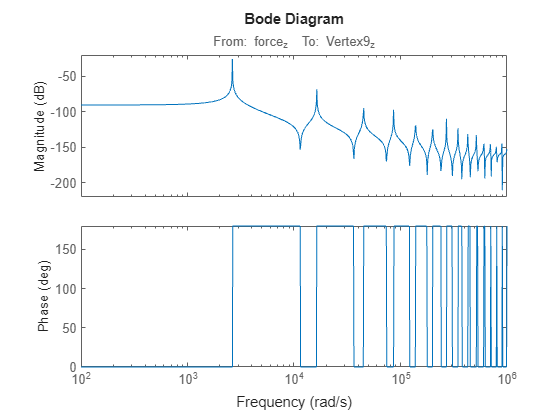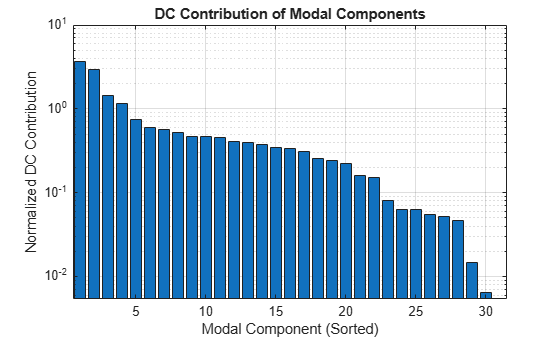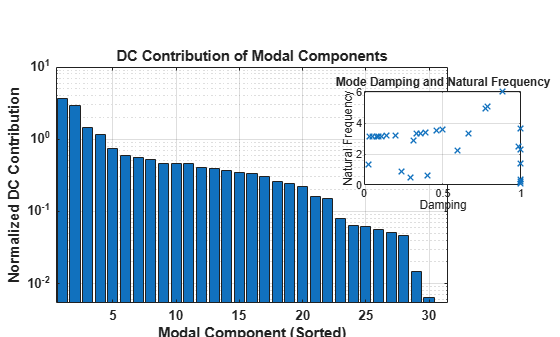# view

Plot mode information when using modal truncation method

Since R2023b

## Syntax

``view(R,type)``
``view(R)``
``view(___,Axes=AX)``
``h = view(___)``
``view(R,"-help")``

## Description

Use `view` to graphically analyze the model and select a model order reduction criteria from a model order reduction task created using `reducespec`. For `ModalTruncation` and `SparseModalTruncation` objects, you can visualize modes (poles) based on their locations, damping and natural frequencies, or normalized DC contributions. For the full workflow, see Task-Based Model Order Reduction Workflow.

example

````view(R,type)` creates a plot that helps you select the modal content of the reduced model. `R` specifies the model order reduction (MOR) specification object. Use the `type` argument to specify these plot types.`"mode"` — Mode locations`"damp"` — Mode damping and natural frequencies`"contrib"` — Bar chart of normalized DC contributions```
````view(R)` plots the default plot type for the model order reduction algorithm of `R`. For the modal truncation method, this syntax plots the mode locations.```

example

````view(___,Axes=AX)` plots on the `Axes` object in the current figure with the handle `AX`. Use this input argument after any of the input argument combinations in the previous syntaxes. For more information about customizing axes, see ```Axes Properties```.```

example

````h = view(___)` returns a plot handle `h`. Use `h` to modify properties of the plot after creating it.```
````view(R,"-help")` returns help specific to the model order specification object `R`. The returned help shows plot types and syntaxes applicable to `R`.```

## Examples

collapse all

This example shows how to obtain a reduced-order model of a structural beam using the modal truncation method. For this example, consider a SISO sparse state-space model of a cantilever beam. This example uses the linearized model from the Linear Analysis of Cantilever Beam example.

```load linBeam.mat size(sys)```
```Sparse second-order model with 1 outputs, 1 inputs, and 3303 degrees of freedom. ```

Plot the Bode response.

`bode(sys,w)`Create a model order reduction task.

`R = reducespec(sys,"modal");`

Set the `Focus` option to compute the eigenvalues only in the specified frequency range.

`R.Options.Focus = [0 5e5];`

Analyze the model and compute the derived information.

```R = process(R); size(R.Mode)```
```ans = 1×2 26 1 ```

The original model contains 26 modes. View the DC contribution of the modal components using the `view` function.

`view(R,"contrib")`Discard the modes with smaller contributions. For this example, discard all modes with DC contribution smaller than `1e-6`. This results in a reduced model with 12 modes.

`[rsys,info] = getrom(R,MinDC=1e-6);`

Plot the bode responses.

`bode(sys,rsys,w)`The reduced-order model provides a good approximation for the original sparse model.

This example shows how customize the plots of modal components obtained using the `view` function.

For this example, create a model order reduction specification for an LTI model using the modal truncation method. Generate a random discrete-time state-space model with 40 states.

```rng(0) sys = drss(40);```

Create a specification object.

`R = reducespec(sys,"modal");`

Visualize the DC contributions of the modes.

`view(R,"contrib")`To customize the plots for the modal truncation method, you can use an `Axes` object.

```figure ax1 = axes('Position',[0.1 0.1 0.7 0.7]); ax2 = axes('Position',[0.65 0.45 0.28 0.28]); h1 = view(R,"contrib",Axes=ax1); h2 = view(R,"damp",Axes=ax2);```

To further customize the plots, use the plot handles to set the options.

```h2.YScale = "linear"; h1.FontWeight = "bold";```## Input Arguments

collapse all

Model order reduction specification object created using `reducespec`, specified as a `ModalTruncation` or `SparseModalTruncation` object.

Plot type, specified as one of the following.

• `"mode"` — Mode locations

• `"damp"` — Mode damping and natural frequencies

• `"contrib"` — Bar chart of normalized DC contributions

If you do not specify this argument, the function plots the mode locations of the original system `sys`.

## Output Arguments

collapse all

Plot handle object, returned as an `Axes` object.

Use dot notation with this handle to customize the plot. For example, `h.YScale = "Linear"`. For a list of available options, see ```Axes Properties```.

## Version History

Introduced in R2023b